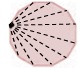Polygons MathBitsNotebook.com Terms of Use   Contact Person: Donna Roberts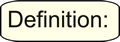A polygon is a 2-dimensional closed figure that is the union of line segments in a plane. A polygon has three or more sides.
 You already have a good understanding of 3-sided and 4-sided polygons from your previous studies of geometry. 3-sided polygons - triangles - all types (acute, right, obtuse, equiangular, scalene, isosceles, equilateral) 4-sided polygons - quadrilaterals - all types (kite, rhombus, square, trapezoid, parallelogram, rectangle) We will now enhance these previous concepts by adding more "sides" to the figures, as well as adding some new information.Classifications of PolygonsPolygons can be classified as either regular or irregular (meaning not regular).A regular polygon is a polygon in which all of the sides are of equal length and all of the angles are of equal measure. It is both equilateral and equiangular.

You have already been working with some regular polygons.
 A regular triangle is an equilateral triangle (also called an equiangular triangle).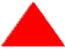All of its sides are the same length. All of its angles are the same measure (60º). A regular quadrilateral is a square.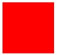All of its sides are the same length. All of its angles are the same measure (90º).

 In a regular polygon, all of its interior angles are of the same measure and all of its sides are the same length.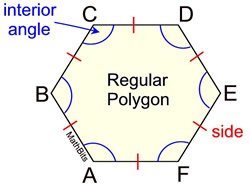Regular Hexagon In an irregular polygon, the interior angles may be of different measures and the sides may be different lengths.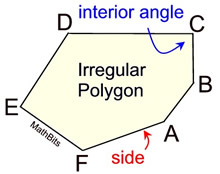Irregular Hexagon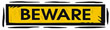The term "polygon" refers to a type of figure which may be regular or irregular. Do NOT assume "polygon" means "regular polygon". If a polygon is a regular polygon, it will be stated as such.Parts of Regular Polygons

 Regular Pentagon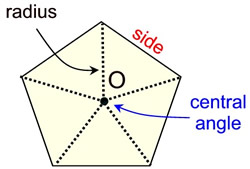The center, (O), of a regular polygon, is a point in the interior of the polygon equidistant from each vertex. It is also the center of the inscribed and circumscribed circles. The radius is the distance from the center to any vertex. The central angle of a regular polygon is formed by connecting the center to two adjacent vertices. All central angles are of equal measure in a regular polygon. Irregular polygons are not described as having a "center" which is equidistant from each vertex. As such, an irregular polygon does not have central angles or radii. An irregular polygon does, however, have a center of gravity or a centroid.Types of Polygons

The table below shows some of the more commonly used polygons.
Do NOT assume that the diagrams in the "Figure" column are "regular" polygons.
Polygon
Figure
Sides
Angles
Diagonals
# of Triangles
from 1 vertex
Triangle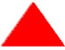3
3
0
 1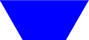4
4
2
 2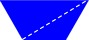Pentagon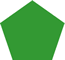5
5
5
 3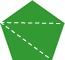Hexagon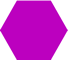6
6
9
 4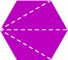Heptagon or Septagon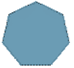7
7
14
 5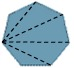Octagon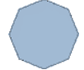8
8
20
 6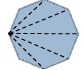Nonagon or Novagon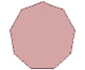9
9
27
 7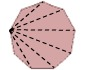Decagon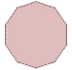10
10
35
 8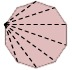Dodecagon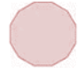12
12
54
 10